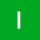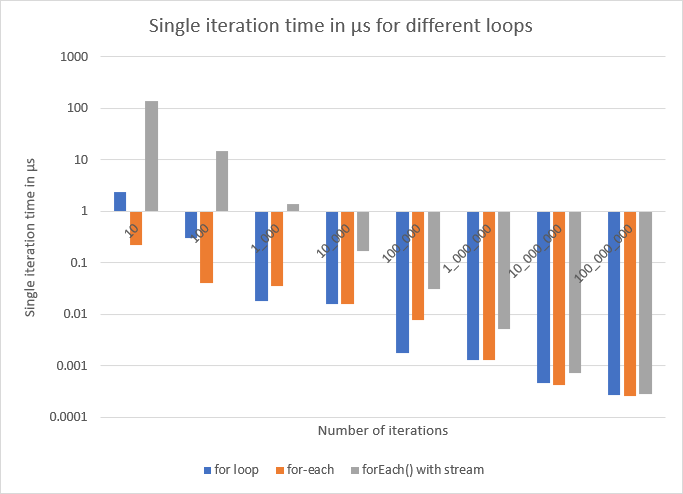Home Communities
IT Knowledge
Inspiration
Languages
EN

# Java - for, for-each and forEach performance test

9 points
Created by:illona
346

In this article, I would like to show results from simple performance tests for different types of `for` loop made on int array in Java.

Tests show what loop type is faster in different cases.Single iteration time in μs for different loops (for loop, for-each, forEach() with stream).

Below test conclusions:

• `for` loop is fastest in many cases,
• time necessary to iterate over array using different for-s going to be similar when array sizes are bigger,
• in some cases `for-each` can be faster, what is visible when array sizes are bigger (for small arrays it is visible mostly).

Used Java: Amazon Corretto, x64, JDK 1.8.0_292

Used OS: Windows 10 x64

Used PC:

• Ryzen 9 5900x
• DRR 4 (2x 32GB)
• Samsung SSD M.2 970 EVO (1TB)
• GeForce GTX 970 (4GB RAM)

Total times needed to iterate over the array:

 `for` type `for` time Array size `for` loop 23.30 μs 10 `for-each` 2.200 μs 10 `forEach()` with stream 1.370 ms 10 `for` loop 29.90 μs 100 `for-each` 4.000 μs 100 `forEach()` with stream 1.457 ms 100 `for` loop 18.20 μs 1_000 `for-each` 36.40 μs 1_000 `forEach()` with stream 1.407 ms 1_000 `for` loop 157.8 μs 10_000 `for-each` 159.4 μs 10_000 `forEach()` with stream 1.667 ms 10_000 `for` loop 176.7 μs 100_000 `for-each` 790.2 μs 100_000 `forEach()` with stream 3.064 ms 100_000 `for` loop 1.282 ms 1_000_000 `for-each` 1.303 ms 1_000_000 `forEach()` with stream 5.150 ms 1_000_000 `for` loop 4.586 ms 10_000_000 `for-each` 4.272 ms 10_000_000 `forEach()` with stream 7.201 ms 10_000_000 `for` loop 27.58 ms 100_000_000 `for-each` 25.87 ms 100_000_000 `forEach()` with stream 28.65 ms 100_000_000

Used source code:

``````import com.google.common.base.Stopwatch;

import java.util.Arrays;
import java.util.function.IntConsumer;

public class Example {

public static void main(String[] args) {

int size = 1_000_000; // change it to your size

int[] array = new int[size];
for (int i = 0; i < array.length; i++) {
array[i] = i;
}

// for loop
{
int sum = 0;

Stopwatch stopwatch = Stopwatch.createStarted();
for (int i = 0; i < array.length; i++) {
sum += array[i];
}
System.out.println(sum + " in " + stopwatch.stop());
}

// for-each loop
{
int sum = 0;

Stopwatch stopwatch = Stopwatch.createStarted();
for (int value : array) {
sum += value;
}
System.out.println(sum + " in " + stopwatch.stop());
}

// forEach method with stream
{

Stopwatch stopwatch = Stopwatch.createStarted();
Arrays.stream(array).forEach(adder); // or just Arrays.stream(array).forEach(value -> { ... });
System.out.println(adder.getSum() + " in " + stopwatch.stop());
}
}

private static class IntAdder implements IntConsumer {

private int sum = 0;

public int getSum() {
return this.sum;
}

@Override
public void accept(int value) {
this.sum += value;
}
}
}``````

`Stopwatch` class from:

``````<!-- https://mvnrepository.com/artifact/com.google.guava/guava -->
<dependency>
<artifactId>guava</artifactId>
<version>27.0.1-jre</version>
</dependency>``````

## Alternative test source code

Alternatively, we can use the following test that repeats same tests few times dividing time by tests amount:

``````import com.google.common.base.Stopwatch;

import java.util.Arrays;
import java.util.concurrent.TimeUnit;
import java.util.function.IntConsumer;

public class Example {

public static void main(String[] args) throws InterruptedException {

int repeatsCount = 1000;
int arraySize = 1_000_000;

TimeUnit resultUnit = TimeUnit.MICROSECONDS;

int[] array = new int[arraySize];
for (int i = 0; i < array.length; i++) {
array[i] = i;
}

int sum1 = 0;
int sum2 = 0;

Stopwatch stopwatch1 = Stopwatch.createUnstarted();
Stopwatch stopwatch2 = Stopwatch.createUnstarted();
Stopwatch stopwatch3 = Stopwatch.createUnstarted();

for (int j = 0; j < repeatsCount; ++j) {

// for loop

stopwatch1.start();
for (int i = 0; i < array.length; i++) {
sum1 += array[i];
}
stopwatch1.stop();

// for-each loop

stopwatch2.start();
for (int value : array) {
sum2 += value;
}
stopwatch2.stop();

// forEach method with stream

stopwatch3.start();
Arrays.stream(array).forEach(adder3); // or just Arrays.stream(array).forEach(value -> { ... });
stopwatch3.stop();
}

double time1 = (double)stopwatch1.elapsed(resultUnit) / repeatsCount;
double time2 = (double)stopwatch2.elapsed(resultUnit) / repeatsCount;
double time3 = (double)stopwatch3.elapsed(resultUnit) / repeatsCount;

String unitAbbreviation = renderUnit(resultUnit);

System.out.println("time: " + time1 + " " + unitAbbreviation + ", sum: " + sum1 + " - for loop");
System.out.println("time: " + time2 + " " + unitAbbreviation + ", sum: " + sum2 + " - for-each loop");
System.out.println("time: " + time3 + " " + unitAbbreviation + ", sum: " + sum3 + " - forEach method with stream");
}

private static class IntAdder implements IntConsumer {

private int sum = 0;

public int getSum() {
return this.sum;
}

@Override
public void accept(int value) {
this.sum += value;
}
}

private static String renderUnit(TimeUnit unit) {

switch (unit) {
case NANOSECONDS:
return "ns";
case MICROSECONDS:
return "\u03bcs"; // μs
case MILLISECONDS:
return "ms";
case SECONDS:
return "s";
case MINUTES:
return "min";
case HOURS:
return "h";
case DAYS:
return "d";
default:
throw new AssertionError();
}
}
}``````

1. Java - iterate over array of strings